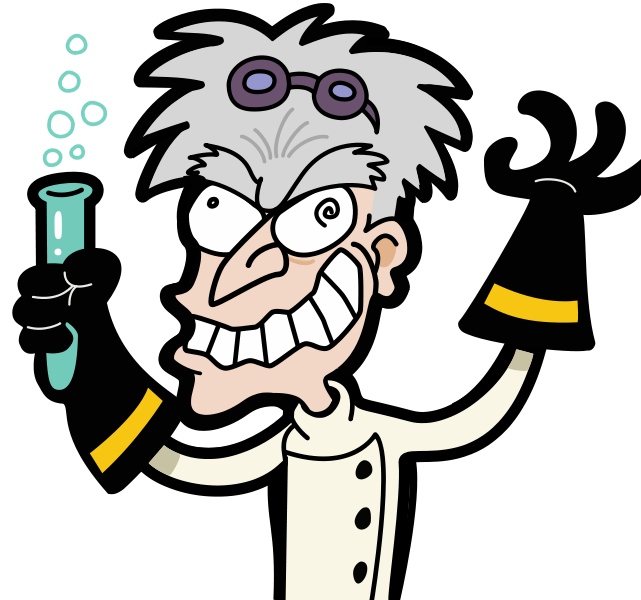## Daily Puzzle Bytes

A new puzzle everyday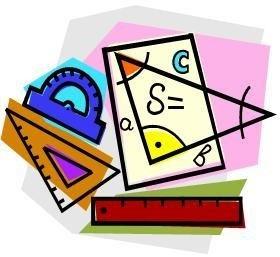#### Puzzle 14

Which letter replaces the question mark and completes the puzzle?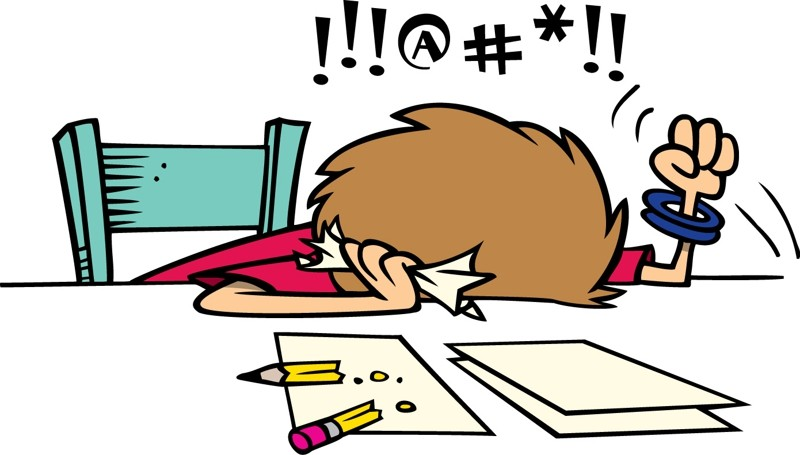#### Puzzle 13

I have only nickels, dimes, and quarters and have at least one of each type of coin. The total number of coins I have is fifteen and the total value of all the coins is \$1.00. How many of each coin do I have?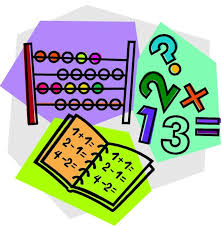#### Puzzle 12

Which number is the odd one out?
9678 4572 5261 5133 3527 6895 7768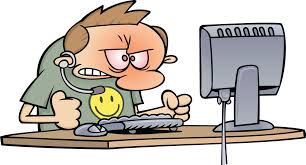#### Puzzle 11

Which number completes the puzzle?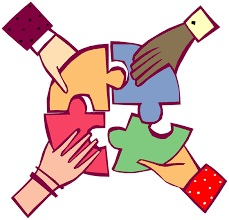#### Puzzle 10

Which letter replaces the question mark to complete this circle?